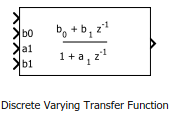# Discrete Varying Transfer Function

Discrete-time transfer function with varying coefficients

• Library:
• Control System Toolbox / Linear Parameter Varying

•## Description

This block implements a discrete-time transfer function with varying coefficients. The instantaneous transfer function is given by:

`$H\left(z\right)=\frac{{b}_{0}+{b}_{1}/z+\cdots +{b}_{N}/{z}^{N}}{1+{a}_{1}/z+\cdots +{a}_{N}/{z}^{N}},$`

where N is number of poles, specified with the Transfer function order parameter. Feed the values of the coefficients a1, …, aN and b0, b1, …, bN to the corresponding block input ports.

Use this block and the other blocks in the Linear Parameter Varying library to implement common control elements with variable parameters or coefficients. For more information, see Model Gain-Scheduled Control Systems in Simulink.

## Ports

### Input

expand all

Transfer function input signal.

Transfer function numerator coefficients. The number of coefficient ports is determined by the Transfer function order parameter.

Transfer function denominator coefficients. The number of coefficient ports is determined by the Transfer function order parameter.

### Output

expand all

Transfer function output signal.

## Parameters

expand all

Transfer function (number of poles), specified as a positive integer. This parameter determines the number of coefficient input ports on the block.

Block sample time, specified as either –1 (inherited sample time) or a positive scalar value.